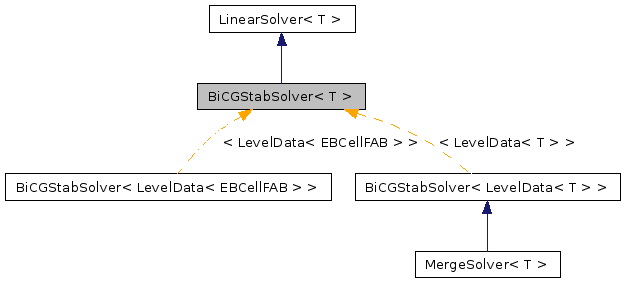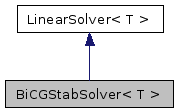# BiCGStabSolver< T > Class Template Reference

`#include <BiCGStabSolver.H>`

Inheritance diagram for BiCGStabSolver< T >:[legend]
Collaboration diagram for BiCGStabSolver< T >:[legend]

## Detailed Description

### template<class T> class BiCGStabSolver< T >

Elliptic solver using the BiCGStab algorithm.

## Public Member Functions

BiCGStabSolver ()
virtual ~BiCGStabSolver ()
virtual void setHomogeneous (bool a_homogeneous)
virtual void define (LinearOp< T > *a_op, bool a_homogeneous)
virtual void solve (T &a_phi, const T &a_rhs)
solve the equation.
virtual void setConvergenceMetrics (Real a_metric, Real a_tolerance)
Set a convergence metric, along with solver tolerance, if desired.

## Public Attributes

bool m_homogeneous
LinearOp< T > * m_op
int m_imax
int m_verbosity
Real m_eps
Real m_reps
Real m_convergenceMetric
Real m_hang
int m_exitStatus
Real m_small
int m_numRestarts
int m_normType

## Constructor & Destructor Documentation

template<class T>
 BiCGStabSolver< T >::BiCGStabSolver ( ) ` [inline]`

template<class T>
 BiCGStabSolver< T >::~BiCGStabSolver ( ) ` [inline, virtual]`

## Member Function Documentation

template<class T>
 virtual void BiCGStabSolver< T >::setHomogeneous ( bool a_homogeneous ) ` [inline, virtual]`

reset whether the solver is homogeneous.

Implements LinearSolver< T >.

Reimplemented in MergeSolver< T >.

template<class T>
 void BiCGStabSolver< T >::define ( LinearOp< T > * a_op, bool a_homogeneous ) ` [inline, virtual]`

define the solver. a_op is the linear operator. a_homogeneous is whether the solver uses homogeneous boundary conditions.

Implements LinearSolver< T >.

Reimplemented in MergeSolver< T >.

References BiCGStabSolver< T >::m_homogeneous, and BiCGStabSolver< T >::m_op.

template<class T>
 void BiCGStabSolver< T >::solve ( T & a_phi, const T & a_rhs ) ` [inline, virtual]`

template<class T>
 void BiCGStabSolver< T >::setConvergenceMetrics ( Real a_metric, Real a_tolerance ) ` [inline, virtual]`

Set a convergence metric, along with solver tolerance, if desired.

Default implementation does nothing, since there are probably cases (liked direct solves), where this has no real meaning.

Reimplemented from LinearSolver< T >.

Reimplemented in MergeSolver< T >.

## Member Data Documentation

template<class T>
 bool BiCGStabSolver< T >::m_homogeneous

public member data: whether the solver is restricted to homogeneous boundary conditions

template<class T>
 LinearOp* BiCGStabSolver< T >::m_op

public member data: operator to solve.

template<class T>
 int BiCGStabSolver< T >::m_imax

public member data: maximum number of iterations

Referenced by BiCGStabSolver< T >::solve().

template<class T>
 int BiCGStabSolver< T >::m_verbosity

public member data: how much screen out put the user wants. set = 0 for no output.

Referenced by MultilevelLinearOp< T >::define(), and BiCGStabSolver< T >::solve().

template<class T>
 Real BiCGStabSolver< T >::m_eps

public member data: solver tolerance

Referenced by BiCGStabSolver< T >::setConvergenceMetrics(), and BiCGStabSolver< T >::solve().

template<class T>
 Real BiCGStabSolver< T >::m_reps

public member data: relative solver tolerance

Referenced by BiCGStabSolver< T >::solve().

template<class T>

public member data: solver convergence metric -- if negative, use initial residual; if positive, then use m_convergenceMetric

Referenced by BiCGStabSolver< T >::setConvergenceMetrics(), and BiCGStabSolver< T >::solve().

template<class T>
 Real BiCGStabSolver< T >::m_hang

public member data: minium norm of solution should change per iterations

Referenced by BiCGStabSolver< T >::solve().

template<class T>
 int BiCGStabSolver< T >::m_exitStatus

public member data: set = -1 if solver exited for an unknown reason set = 1 if solver converged to tolerance set = 2 if rho = 0 set = 3 if max number of restarts was reached

Referenced by BiCGStabSolver< T >::solve().

template<class T>
 Real BiCGStabSolver< T >::m_small

public member data: what the algorithm should consider "close to zero"

Referenced by BiCGStabSolver< T >::solve().

template<class T>
 int BiCGStabSolver< T >::m_numRestarts

public member data: number of times the algorithm can restart

Referenced by BiCGStabSolver< T >::solve().

template<class T>
 int BiCGStabSolver< T >::m_normType

public member data: norm to be used when evaluation convergence. 0 is max norm, 1 is L(1), 2 is L(2) and so on.

Referenced by BiCGStabSolver< T >::solve().

The documentation for this class was generated from the following file:

Generated on Tue Apr 14 14:22:55 2009 for Chombo + EB by1.5.5